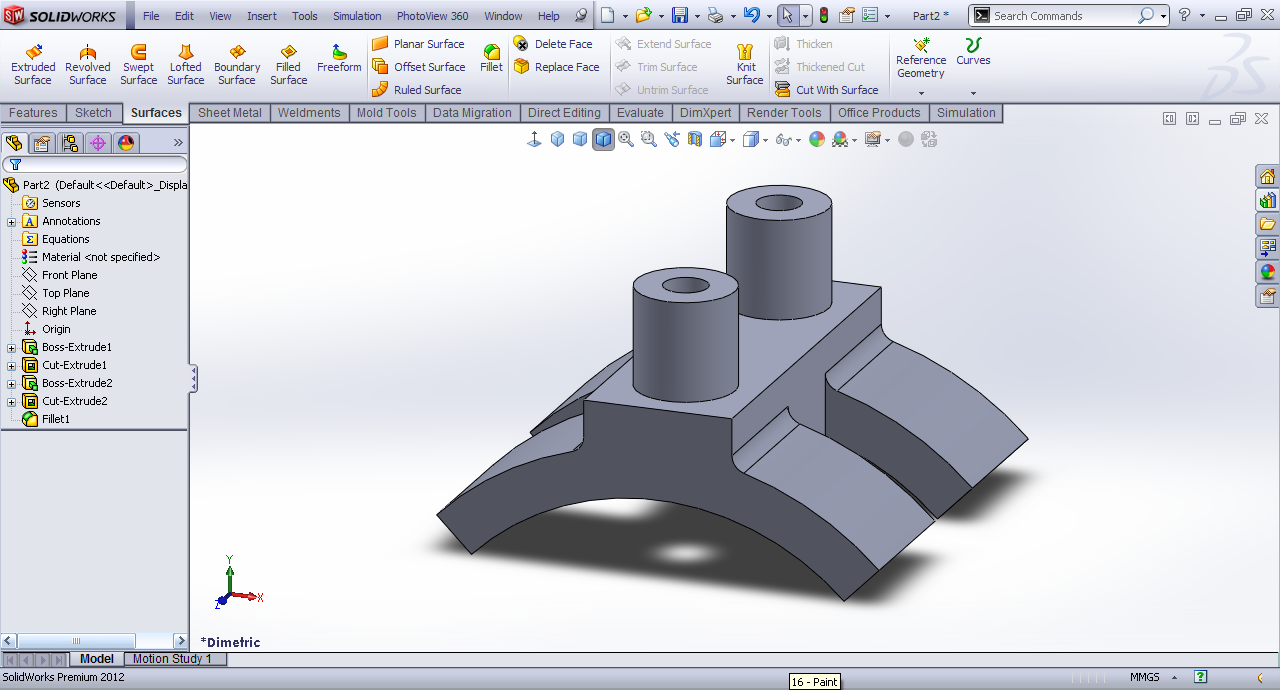# Tutorial - Modeling saddle support in SolidWorks?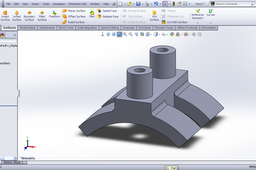Here is the tutorial.

1. ### Step 1:

Front Plane>>Sketch.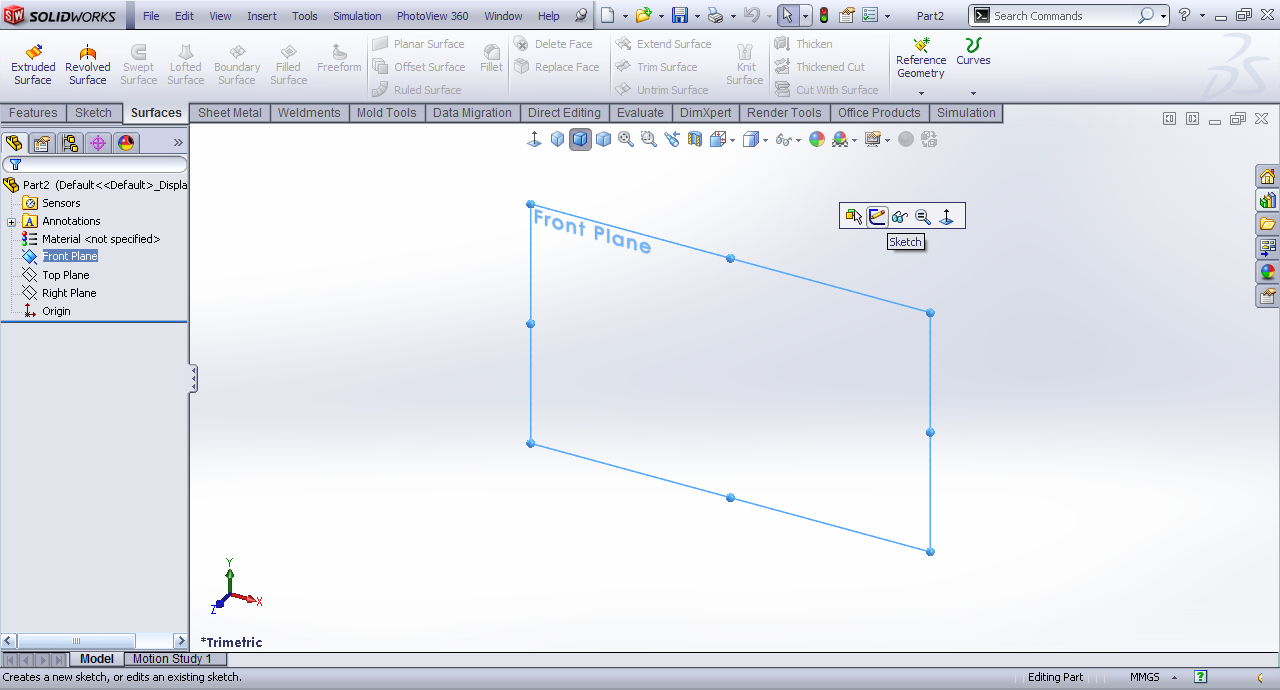2. ### Step 2:

Draw two circles.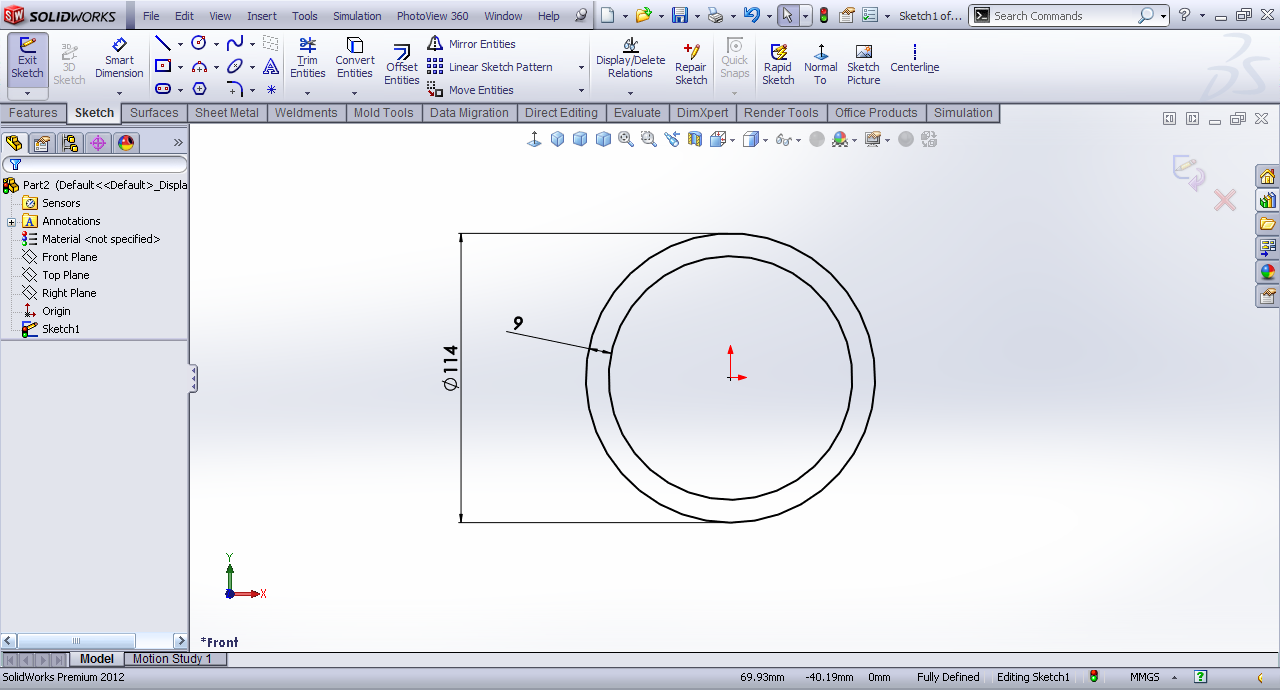3. ### Step 3:

Draw two lines at right angle to each other such that it is at 45º to the vertical center line at origin.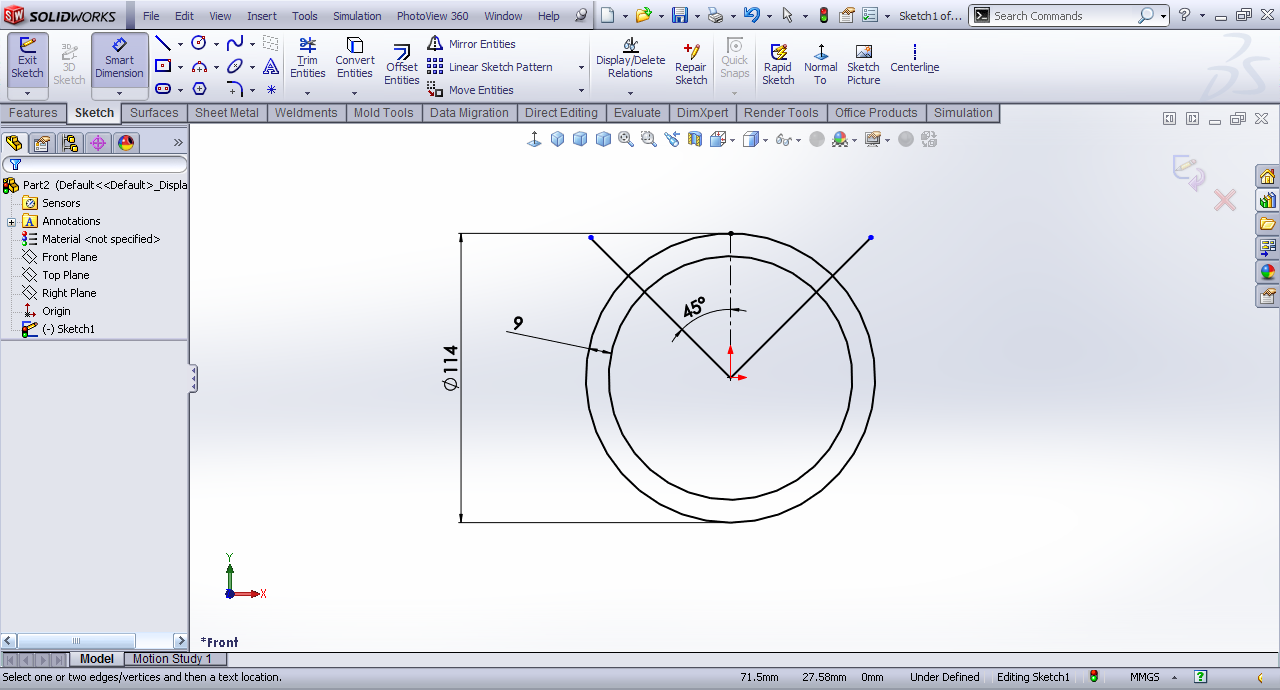4. ### Step 4:

Make a center-point rectangle.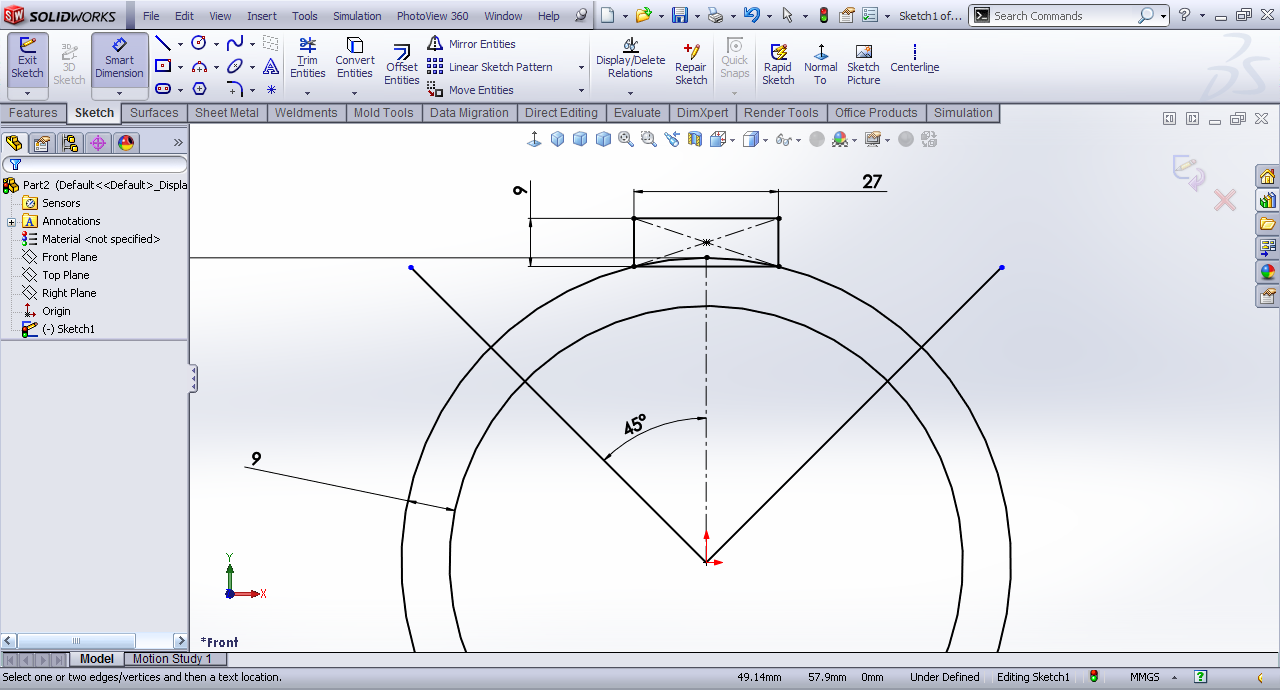5. ### Step 5:

Trim the unncessary entities.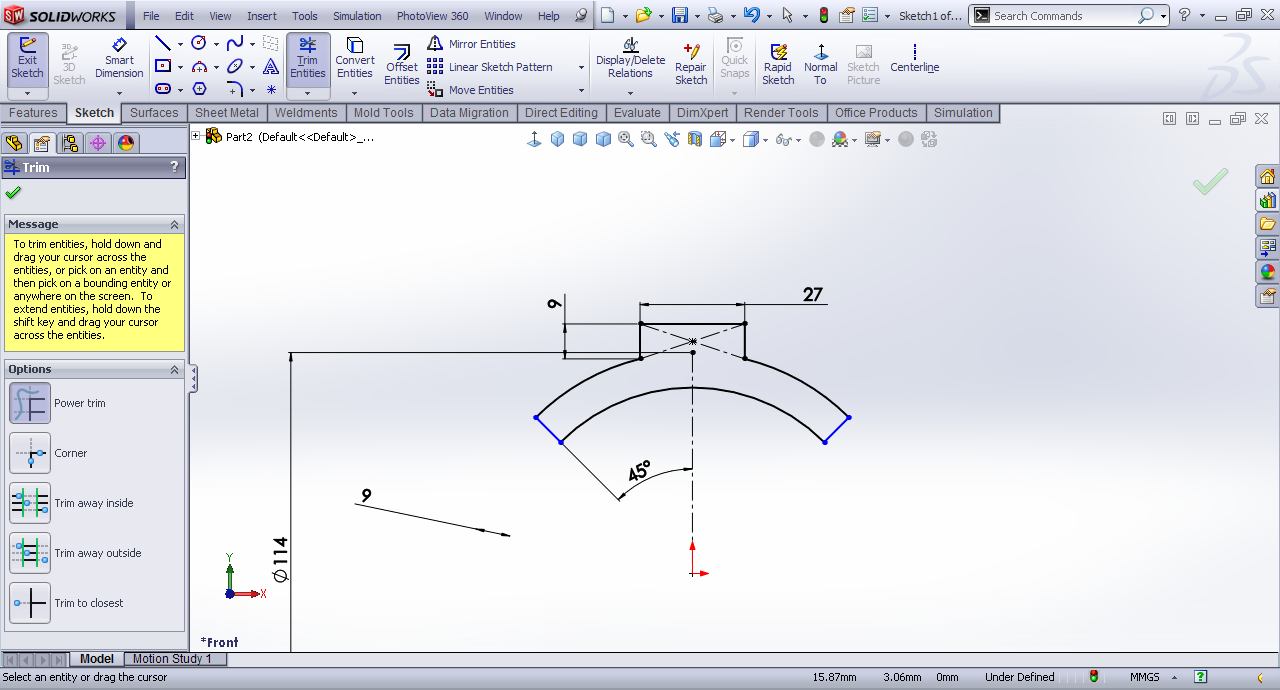6. ### Step 6:

Extrude it mid-plane by 72mm.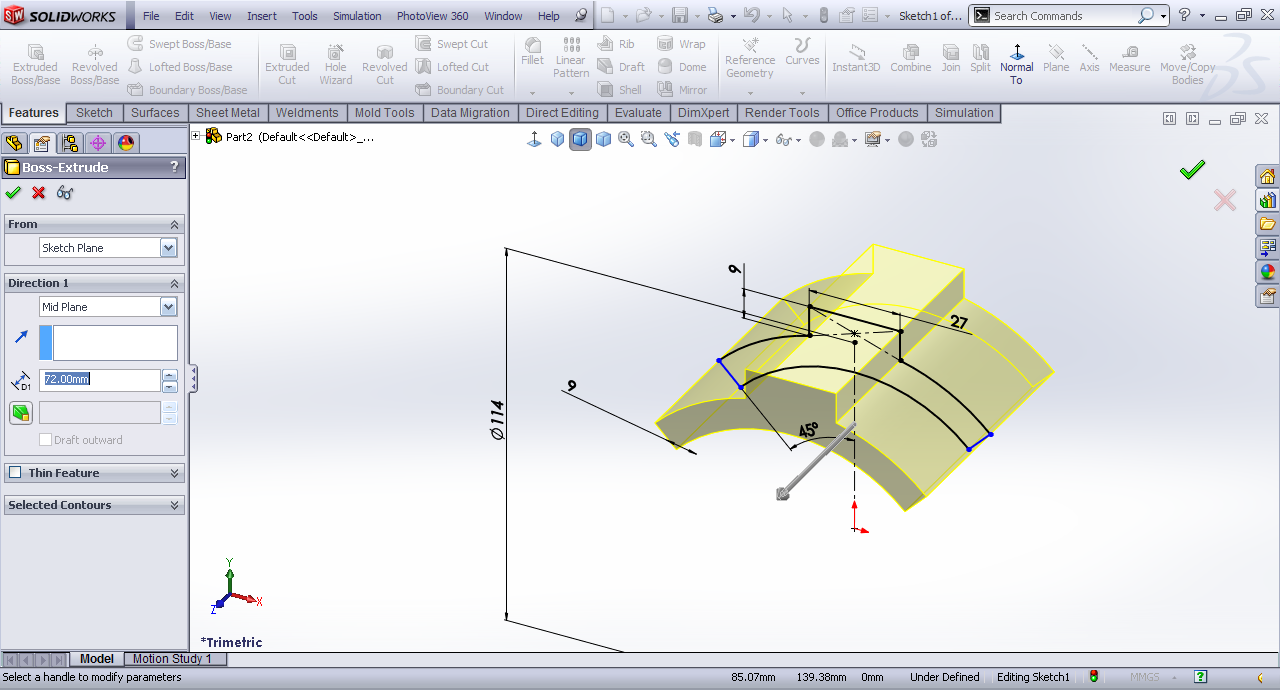7. ### Step 7:

Front plane>>Sketch.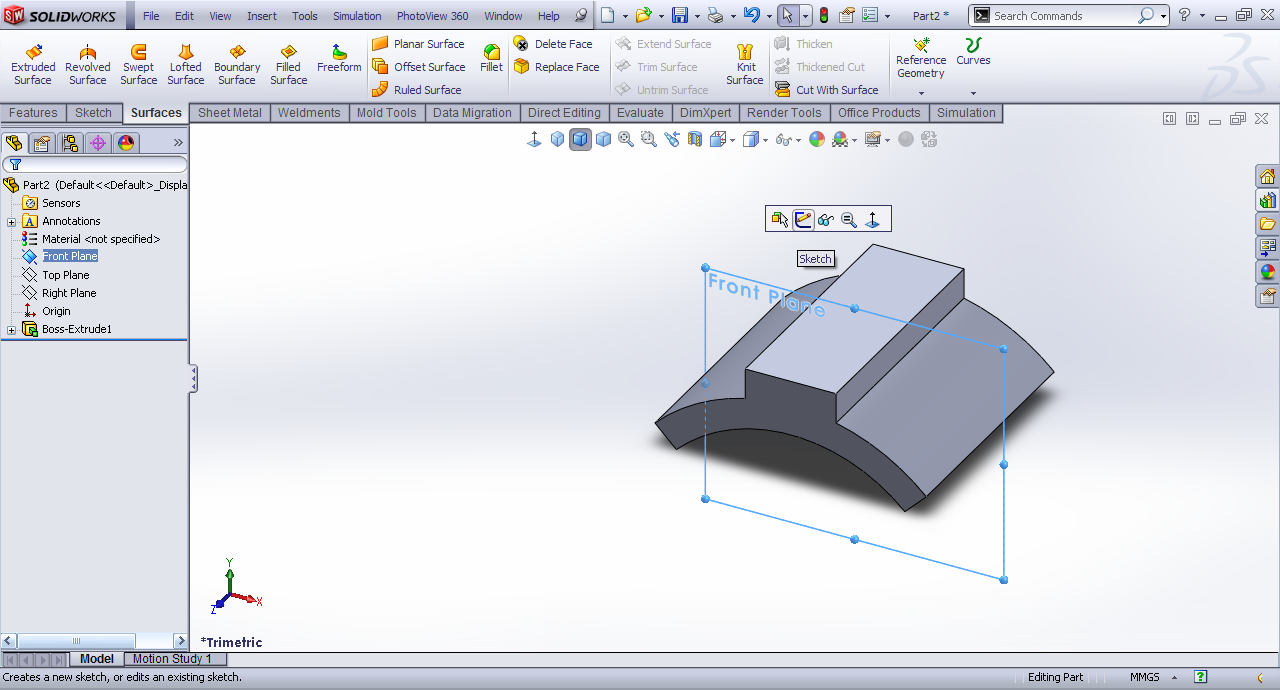8. ### Step 8:

Draw two rectangles like these.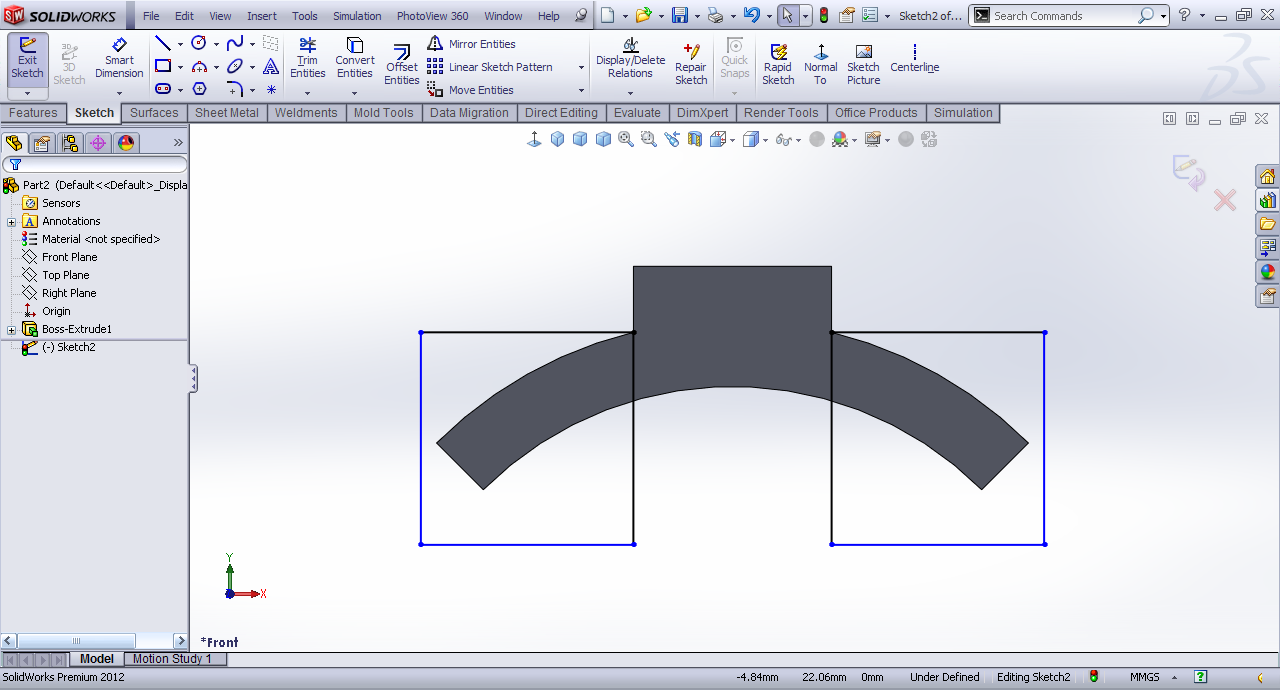9. ### Step 9:

Extruded cut by mid plane.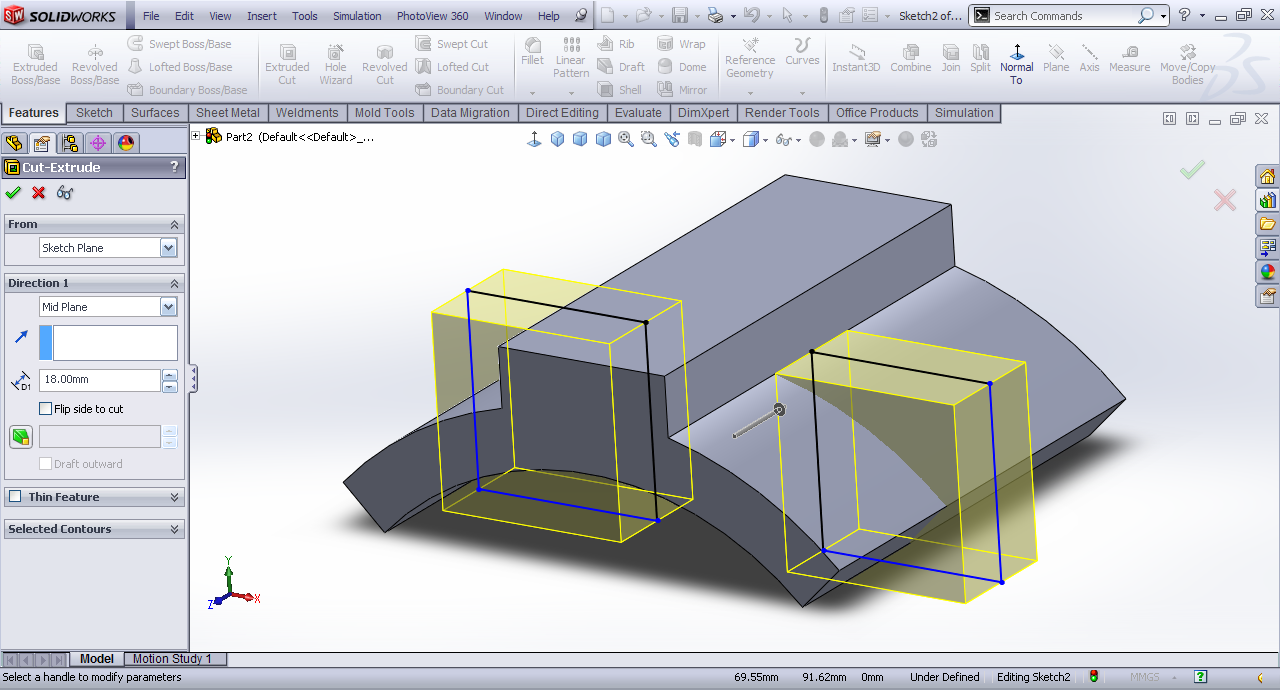10. ### Step 10:

Top face>>Sketch.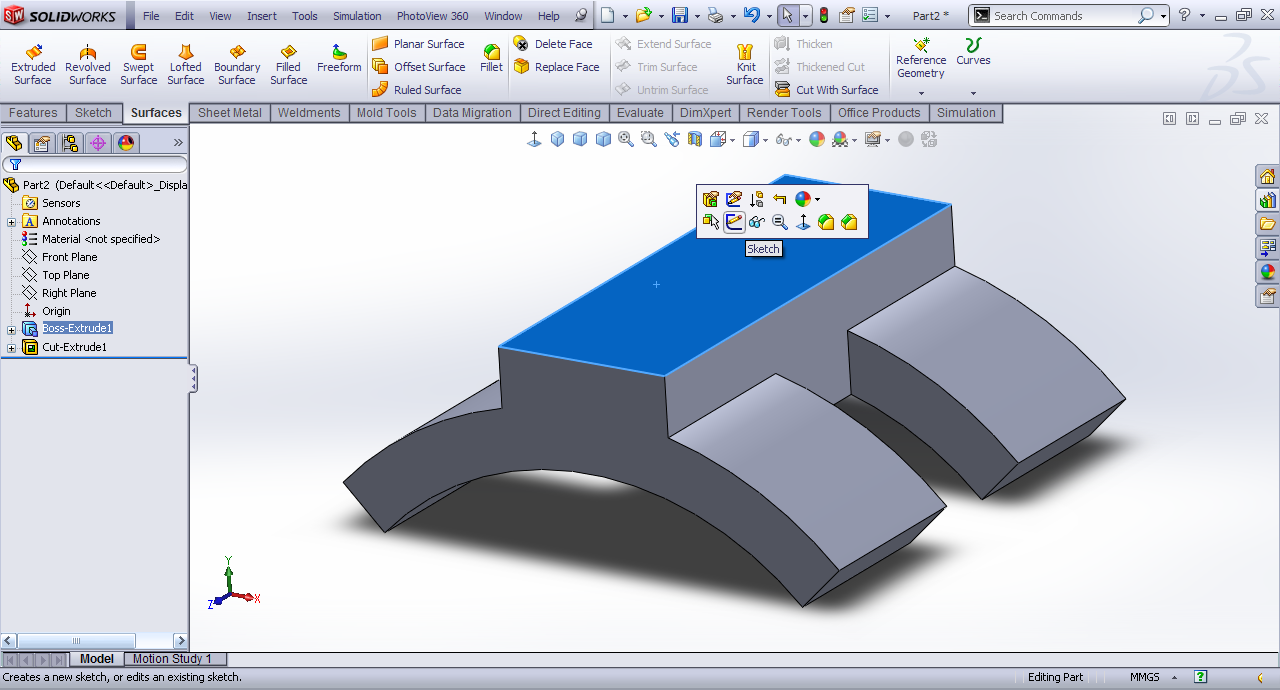11. ### Step 11:

Draw two circles.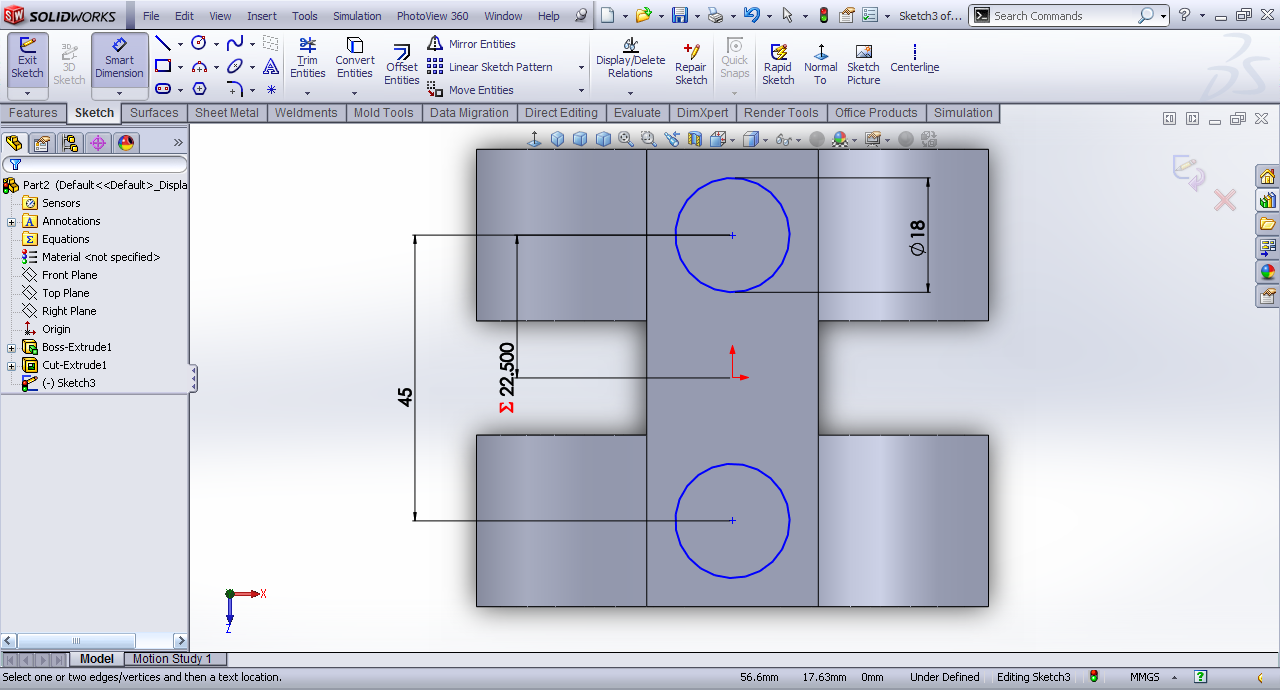12. ### Step 12:

Extrude them.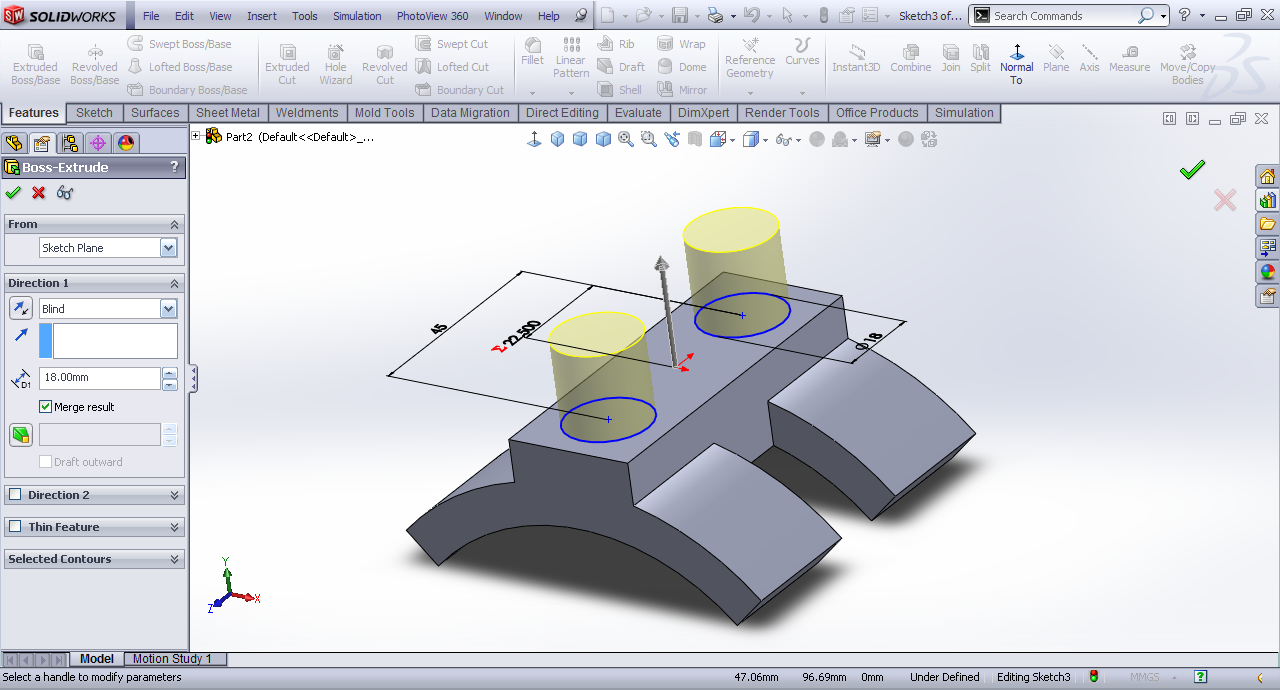13. ### Step 13:

Top face>>Sketch.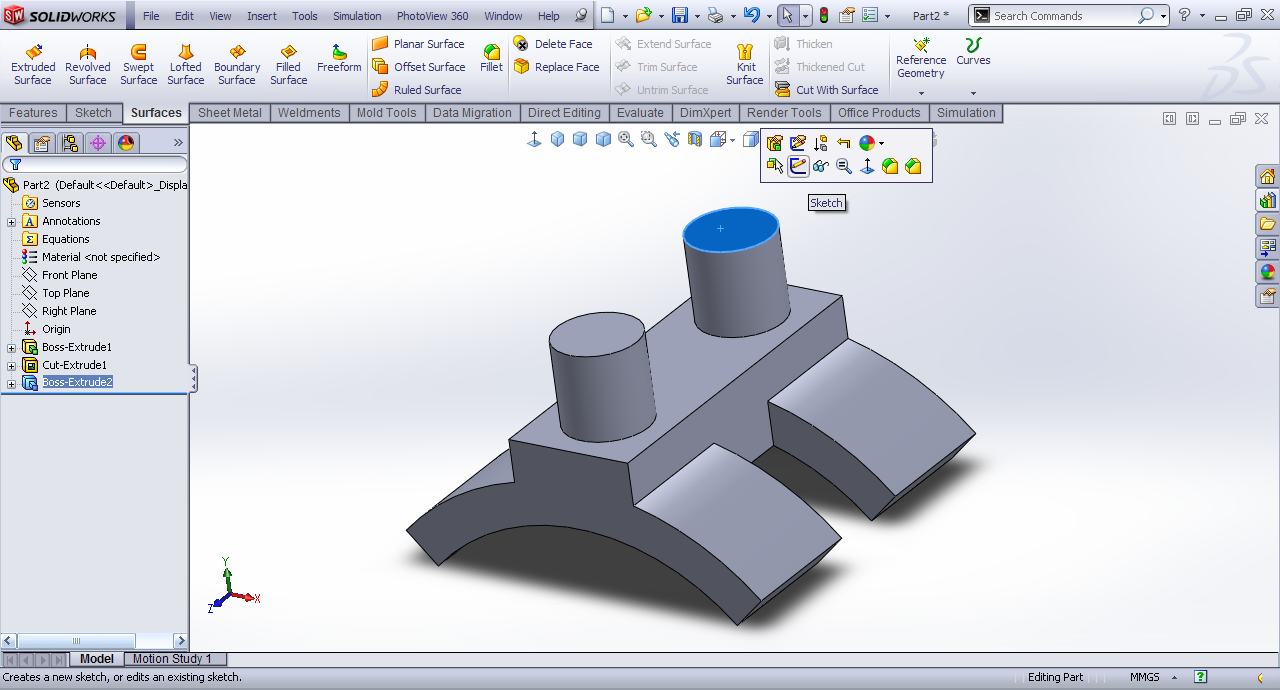14. ### Step 14:

Draw two circles.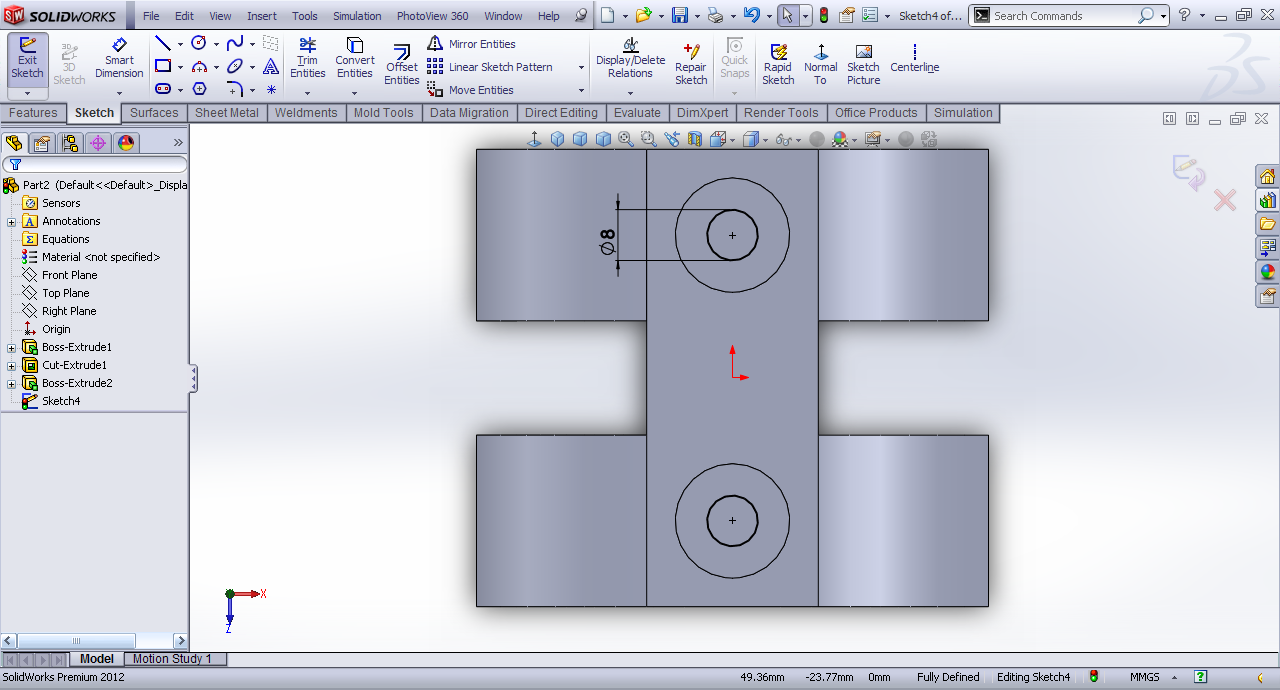15. ### Step 15:

Extruded cut by through all.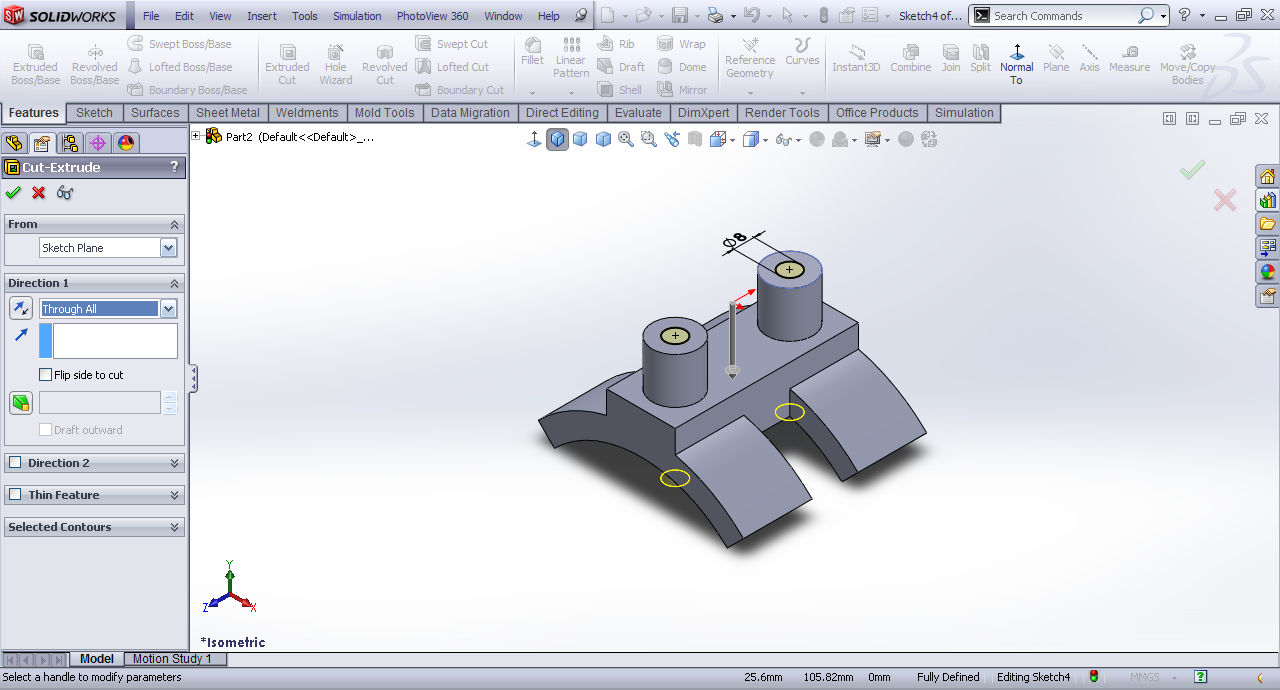16. ### Step 16:

Fillet the rounded edges.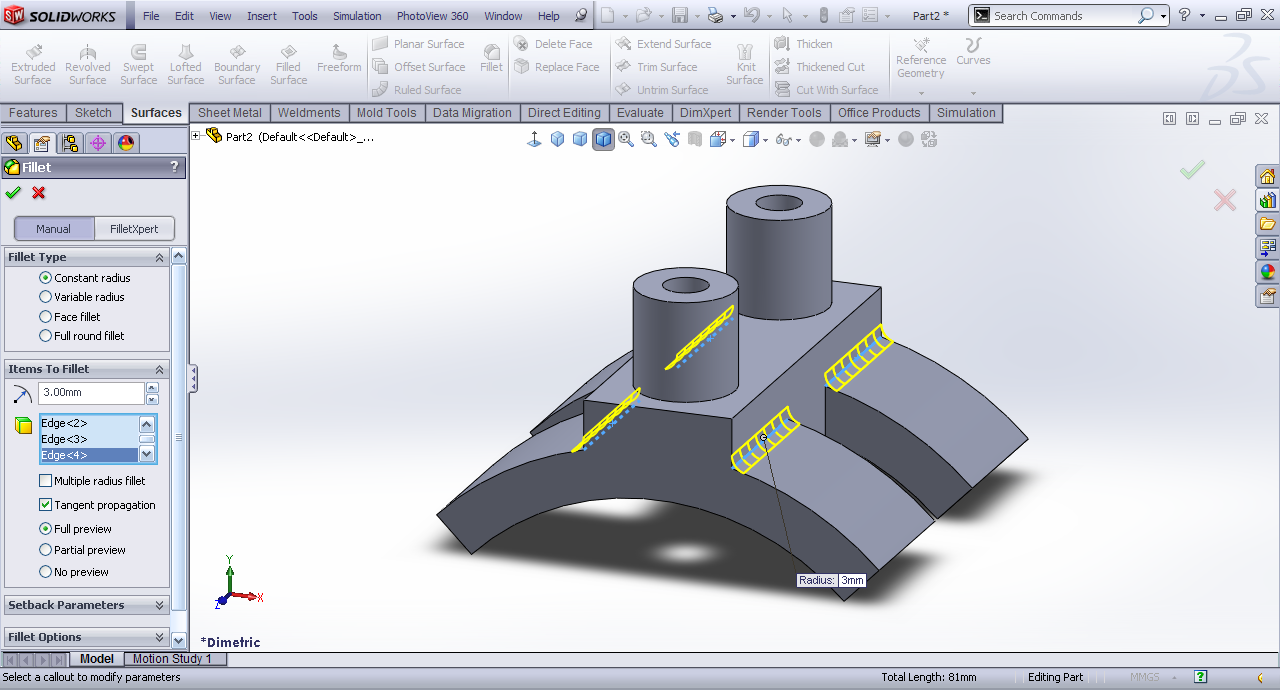17. ### Step 17: